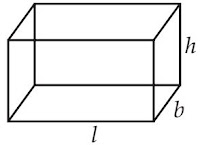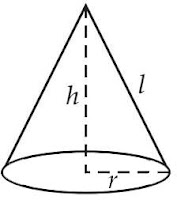## Revision Notes of Chapter 13 Surface Areas and Volumes Class 9th Math

Topics in the Chapter
• Basic Revision
• Formula for Cuboid
• Formula for Cube
• Formula for Right Circular Cylinder
• Formula for Right Circular Cone
• Formula for Sphere
• Formula for Hemisphere
• Tips and Notes
Basics Revision
• The bodies occupying space are called solids, such as a cuboid, a cube, a cylinder, a cone, a sphere, etc. These solids have plane or curved surfaces.
• The outer surface of a cuboid is made up of six rectangles. If we take the length of the cuboid as ℓ, breadth as b and the height as h, then Surface area of a cuboid = 2[lb + bh + hl]
• The cuboid whose length, breadth and height are equal is called a cube. Let each edge of the cube be ‘a’, then Surface area of a cube = 6a2
Formula for CuboidCurved Surface Area for Cuboid or Area of four walls = 2(bh + hl)  = 2(l + b) × h
Total Surface Area for Cuboid = 2(lb + bh + hl)
Volume for Cuboid = l×b×h

Formula for CubeCurved Surface Area for Cube or Area of four walls = 4l2
Total Surface Area for Cube = 6l2
Volume for Cube = l3

Formula for Right Circular CylinderCurved Surface Area for Right Circular Cylinder = 2πrh
Total Surface Area for Right Circular Cylinder = 2πr(r + h)
Volume for Right Circular Cylinder = πr2h

Formula for Right Circular ConeSlant height of right Circular Cone:Curved Surface Area for Right Circular Cone = πrl
Total Surface Area for Right Circular Cone = πr(r + l)
Volume for Right Circular Cone = 1/3 (πr2h)

Formula for SphereCurved Surface Area for Sphere = 2πrh
Total Surface Area for Sphere = 2πr(r + h)
Volume for Sphere = πr2h

Formula for HemisphereCurved Surface Area for Hemiphere = 4πr2
Total Surface Area for Hemiphere = 4πr2
Volume for Hemiphere = 4/3(πr3)

Tips and Notes

• Area × Rate = Cost
• Density = Mass/Volume
• 1 m3 = 1000 litres
• 1 L = 1000 cm2
• Speed = Distance/Time
• 1 km = 1000 m = 105 cm
• 1 km2 = 106 m2
• 1 m = 100 cm
• 1 m2 = 10000 cm2
• 1 km/hr = 5/18 m/sec
• 1 km/hr = 50/3 m/min
• Shape of the river = cuboid